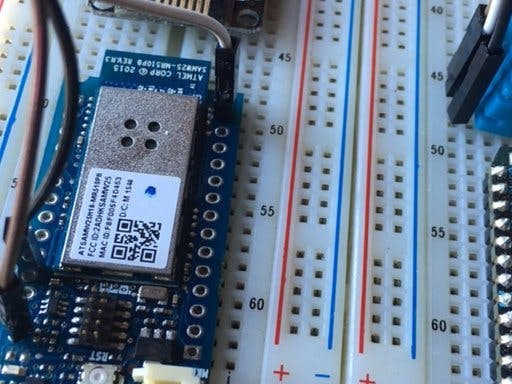# Arduino Voltmeter With OLED Display

Hello !

I’m going to show you how to make a voltmeter* with Arduino UNO, an OLED display, 2 resistors and 9 jumpers for less than 5 minutes. With it you will be able to measure Voltage from 0 to 50V (if you exceed it you may damage your Arduino !). Lets begin !*”A voltmeter is an instrument used for measuring electrical potential difference between two points in an electric circuit” – Wikipedia

## Step 1: Parts and Software

For the voltmeter you will need:

• An Arduino UNO board
• An OLED display – 1.3′ 132×64 with SH1106 controller
• 2 resistors – 10 k and 100k
• 9 jumper wires

You will need the Arduino IDE that you can get from:

https://www.arduino.cc/en/main/software

and the U8glib library for the OLED that you can get from:

or

https://github.com/olikraus/u8glib

## Step 2: Making the Voltmeter

I order to measure voltage we will a build a simple voltage divider, but first place the OLED board on the breadboard. Then connect pin 13 to CLK, pin 11 to DIN, pin 9 to CS, pin 8 to D/C and pin 7 to RES.

Pins: 13, 11, 9 are the SPI Bus of the Arduino UNO board.

Now we build the voltage divider, plug the resistors on the breadboard and connect them for each other, the other side of the 100k resistor connect to ground, the connection between both reistors to analog 0 and the other side of the 10k resistor to the source you wish to measure, like on the schematic above.

After that move to the next step.

## Step 3: Code

#### This Post / Project can also be found using search terms:

• voltmeter oled arduino
• arduino oled 1 3 volt
• arduino voltimetro oled
• oled voltmeter arduino